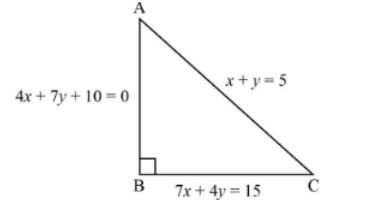QuestionAnswers

# Find the orthocentre of the triangle formed by $4x - 7y + 10 = 0$ , $x + y - 5 = 0$ , $7x + 4y - 15 = 0$ as sides.Verified
91.8k+ views
Hint: We will write the three equations of the lines forming the sides of the triangle. Then from the first equation we will find the value of $y$ in terms of other variables. Then we will substitute the value of $y$ in the other equation. Then the equation will be in the form of one variable which can easily be solved. Then we find the value of $x$ for this $y$ . Since these are the point of intersection of three lines hence it will be orthocentre of the triangle.

The following is the schematic diagram of the triangle.The given lines are $4x - 7y + 10 = 0$ , $x + y - 5 = 0$ , $7x + 4y - 15 = 0$ which are sides of the triangle.
We can name the equations as
$4x - 7y + 10 = 0$ ……(i)
$x + y - 5 = 0$ ……(ii)
$7x + 4y - 15 = 0$ …….(iii)
We will find the slope of line (i),
$4x - 7y + 10 = 0\\ 7y = 4x + 10\\ y = \dfrac{4}{7}x + \dfrac{{10}}{7}$
The above equation is in the form of $y = mx + c$ which is the equation of straight-line having slope $m$ . Hence this equation has slope $\dfrac{4}{7}$ .
We will find the slope of line (iii)
$7x + 4y - 15 = 0\\ 4y = - 7x + 15\\ y = - \dfrac{7}{4}x + \dfrac{{15}}{4}$
The slope of line (iii) is $- \dfrac{7}{4}$ .
Since the product of slope of line (i) and (iii) is $- 1$ . Hence line (i) and (iii) are perpendicular lines. Now to find the orthocentre we will find the point of intersection of these lines. Which will give us an orthocentre.
From equation (i) we can write $y$ as
$4x - 7y + 10 = 0\\ 4x + 10 = 7y\\ y = \dfrac{{4x + 10}}{7}$ ……(iv)
Now we will substitute the value of $y$ in equation (iii) we will get
$7x + 4\left( {\dfrac{{4x + 10}}{7}} \right) - 15 = 0\\ 7x + \dfrac{{16x + 40}}{7} - 15 = 0\\ 49x + 16x + 40 - 105 = 0\\ 65x - 65 = 0\\ 65x = 65\\ x = 1$
We will substitute 1 for $x$ in equation (iv) to find $y$ .
$y = \dfrac{{4\left( 1 \right) + 10}}{7}\\ y = \dfrac{{14}}{7}\\ y = 2$
Hence we can say that $\left( {1,2} \right)$ will be the point of intersection for equation (i) and (iii). Hence we can say that $\left( {1,2} \right)$ is the orthocentre of the triangle.
So, the correct answer is “ $\left( {1,2} \right)$ ”.

Note: The orthocentre is the point at which the altitudes of the three sides of the triangles will intersect. For a right angle triangle the orthocentre can be defined as a point at which the right angle is formed. That’s why we need to find the point of intersection of the lines which are perpendicular in the triangle.